WEST Math: Rational Expressions Chapter Exam

Exam Instructions:

Choose your answers to the questions and click 'Next' to see the next set of questions. You can skip questions if you would like and come back to them later with the yellow "Go To First Skipped Question" button. When you have completed the practice exam, a green submit button will appear. Click it to see your results. Good luck!

Page 1

Question 2 2. Simplify.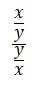Question 4 4. Solve for x.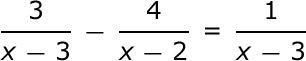Page 2

Question 6 6. Simplify.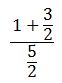Question 7 7. Divide the following expressions: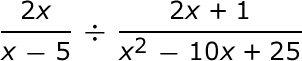Question 8 8. Multiply the following expressions: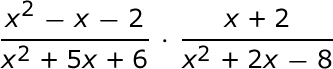Question 9 9. Simplify.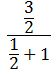Question 10 10. Divide the following expressions: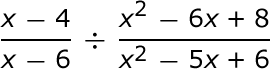Page 3

Question 11 11. What is the horizontal asymptote of the following function?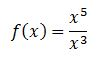Question 15 15. Simplify.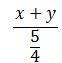Page 4

Question 16 16. Subtract and simplify.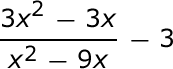Question 17 17. What is the horizontal asymptote of the following function?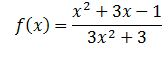Question 18 18. What is(are) the vertical asymptotes of the following function?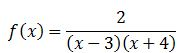Question 19 19. Add and simplify.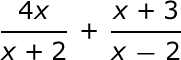Page 5

Question 21 21. Subtract and simplify.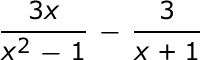Question 22 22. Simplify.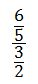Question 23 23. Solve for x.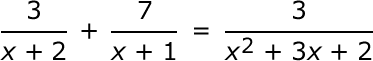Question 24 24. Multiply the following expressions: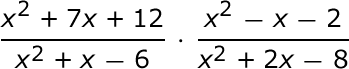Question 25 25. Solve for x.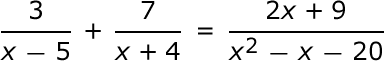Page 6

Question 26 26. Solve the following rational equation.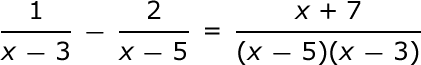Question 27 27. Add and simplify.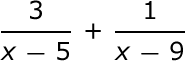Question 28 28. Solve the rational equation.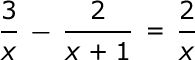Question 29 29. Subtract and simplify.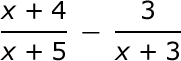WEST Math: Rational Expressions Chapter Exam Instructions

Choose your answers to the questions and click 'Next' to see the next set of questions. You can skip questions if you would like and come back to them later with the yellow "Go To First Skipped Question" button. When you have completed the practice exam, a green submit button will appear. Click it to see your results. Good luck!

Support